Question 7 asks if some gold jewelry is real or not. Can you perform the calculation required?

# Conversion Problems (Metric to Metric)

This Math quiz is called 'Conversion Problems (Metric to Metric)' and it has been written by teachers to help you if you are studying the subject at high school. Playing educational quizzes is a user-friendly way to learn if you are in the 9th or 10th grade - aged 14 to 16.

It costs only \$12.50 per month to play this quiz and over 3,500 others that help you with your school work. You can subscribe on the page at Join Us

So, no imperial measures in this high school Math metric conversion quiz, did you think you were safe? Think again! The metric system is based around a standard base unit (called SI, or Systeme International) for each type of measurement – the meter for length, gram for mass, and liter for volume.

If we want to describe very large or very small measurements, we need to add a prefix to the base unit. You will recognize many of these:

• milli means thousandth (1mm is one thousandth of a meter, or there are 1,000mm in 1m)
• kilo means thousand, so a kilogram is 1,000 grams
• centi, as in centimeter, is one hundredth

Do you know any others? You should be very familiar working between the measures that are common in everyday life.

Volume is the measure that probably causes most problems, particularly when it involves liquids. A key fact to remember is that 1 liter of water weighs 1kg, and a cubic meter is really big! Mark it out on the floor, 1 meter by 1 meter square, then raise it up by a meter. If this cube were filled with water, it would weight a ton (metric of course!).

Question 1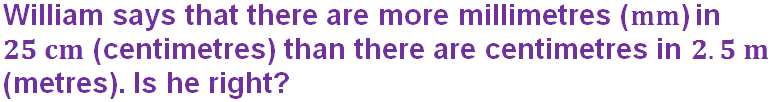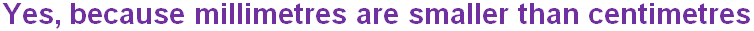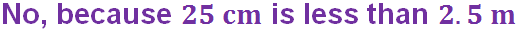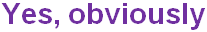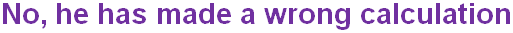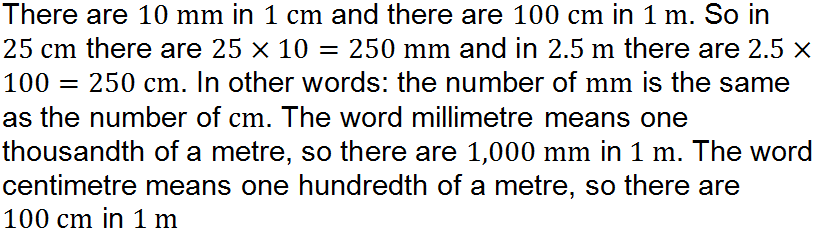Question 2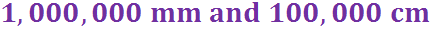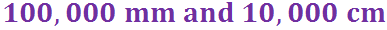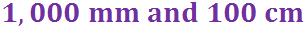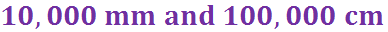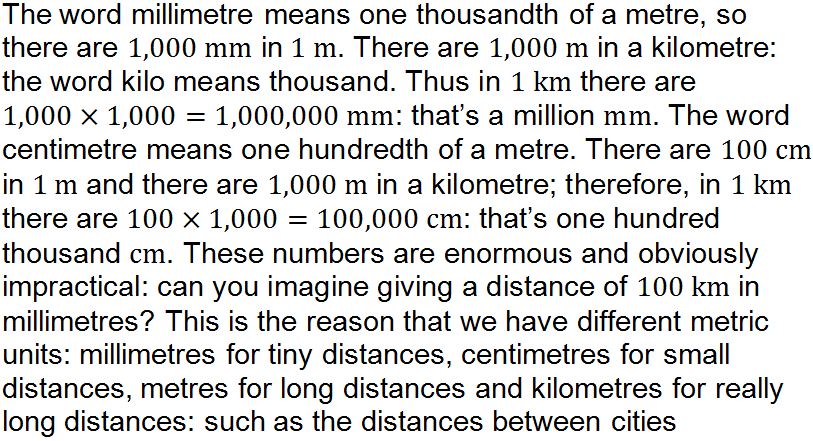Question 3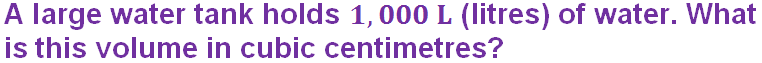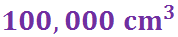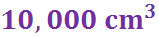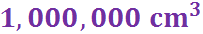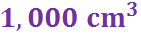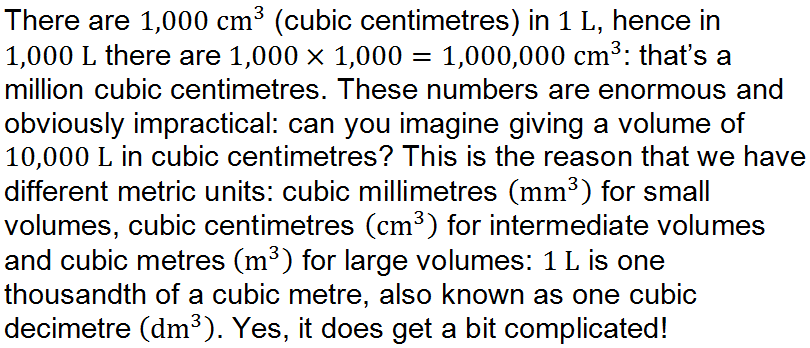Question 4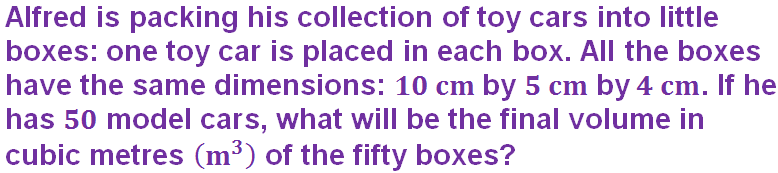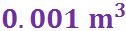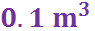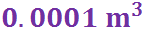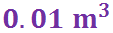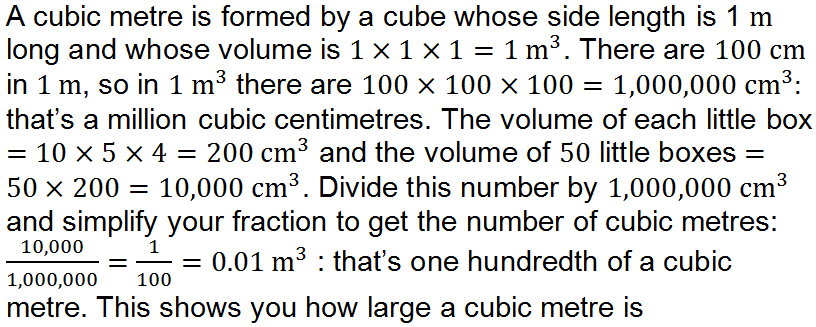Question 5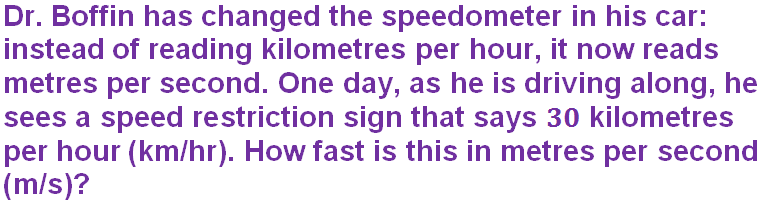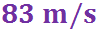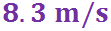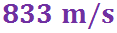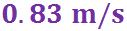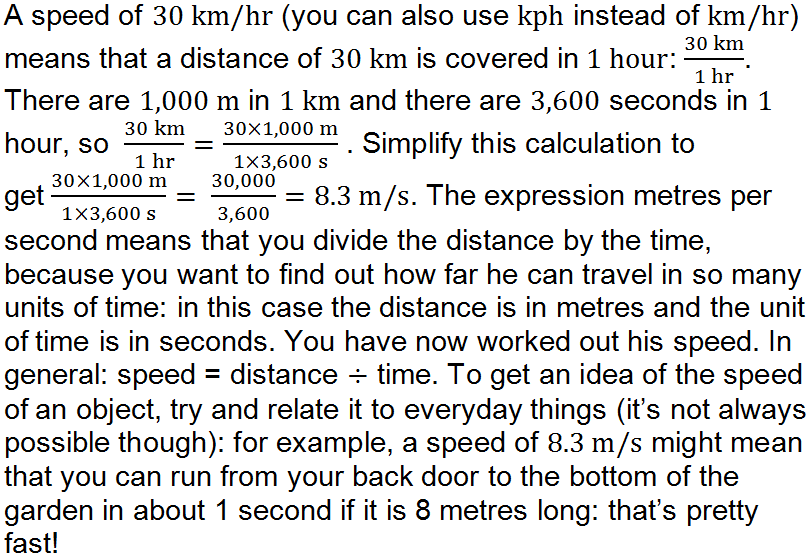Question 6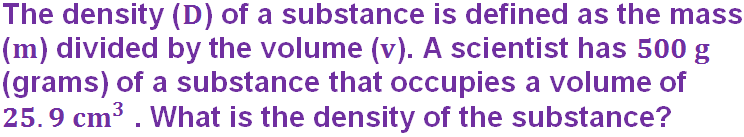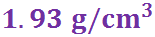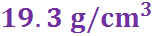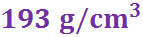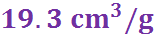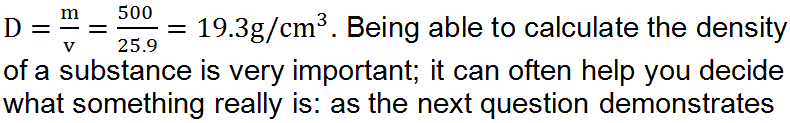Question 7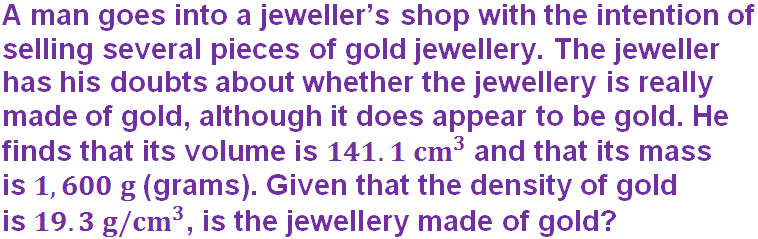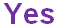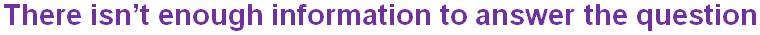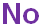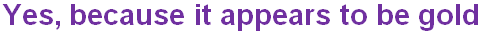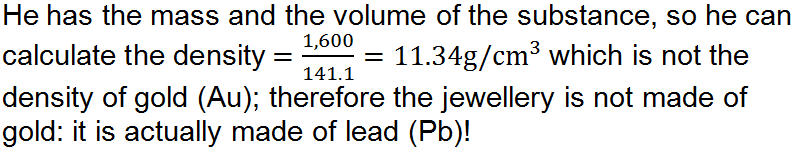Question 8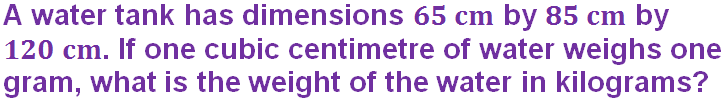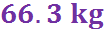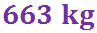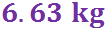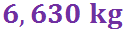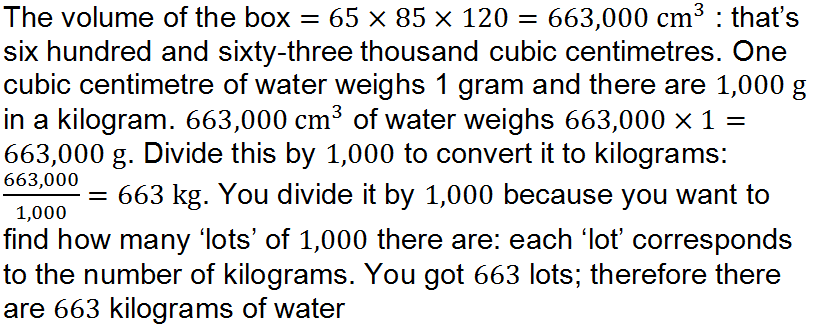Question 9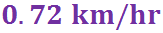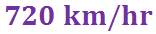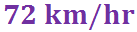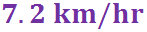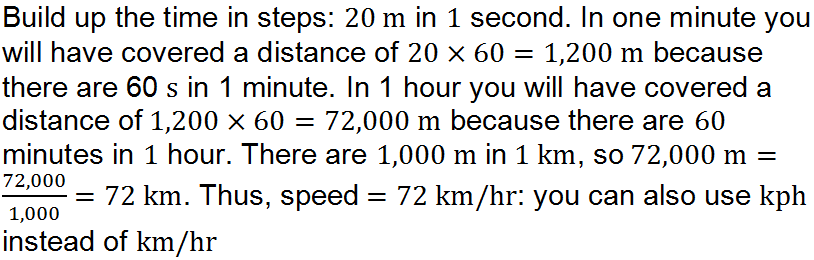Question 10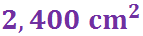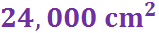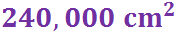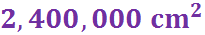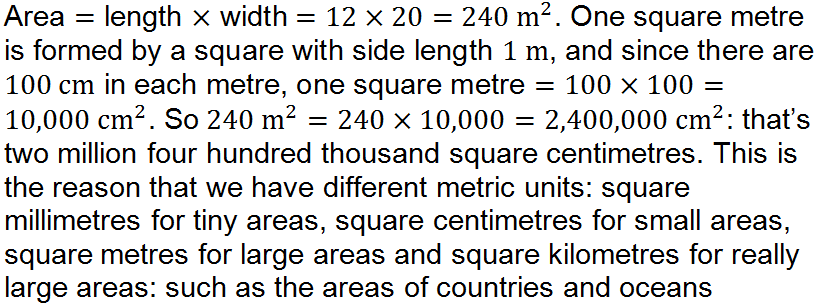Author:  Frank Evans